# Selina Solutions Concise Mathematics Class 6 Chapter 19: Fundamental Operations Exercise 19(C)

Selina Solutions Concise Mathematics Class 6 Chapter 19 Fundamental Operations Exercise 19(C) has accurate answers prepared by experts at BYJU’S, with the intention to improve grasping abilities of students. Multiplication of algebraic expressions is the major concept discussed under this exercise. Students who aim to score well in the annual exam are suggested to solve the textbook questions, using these solutions. To speed up the problem solving abilities, students can make use of Selina Solutions Concise Mathematics Class 6 Chapter 19 Fundamental Operations Exercise 19(C) PDF, from the links which are provided, with a free download option.

## Selina Solutions Concise Mathematics Class 6 Chapter 19: Fundamental Operations Exercise 19(C) Download PDF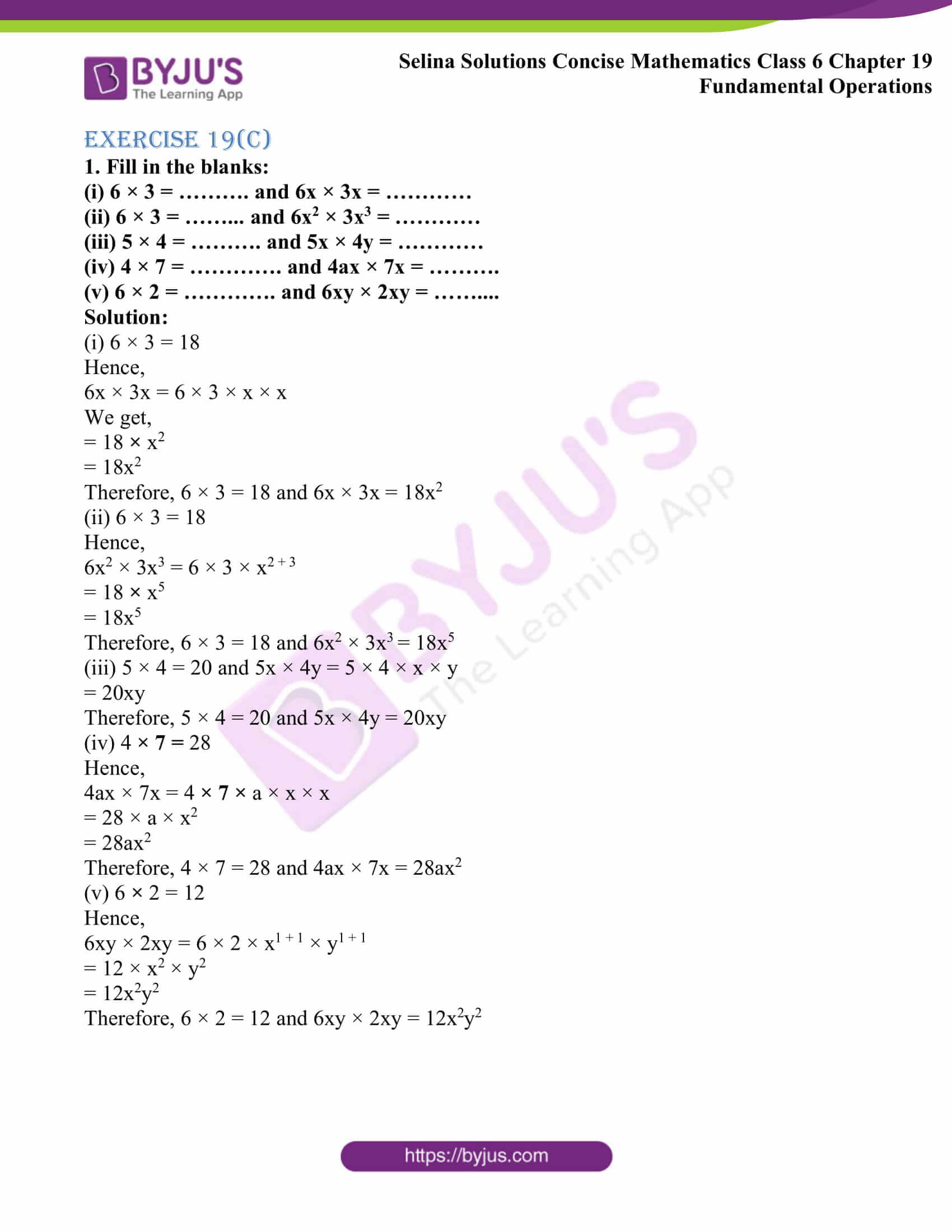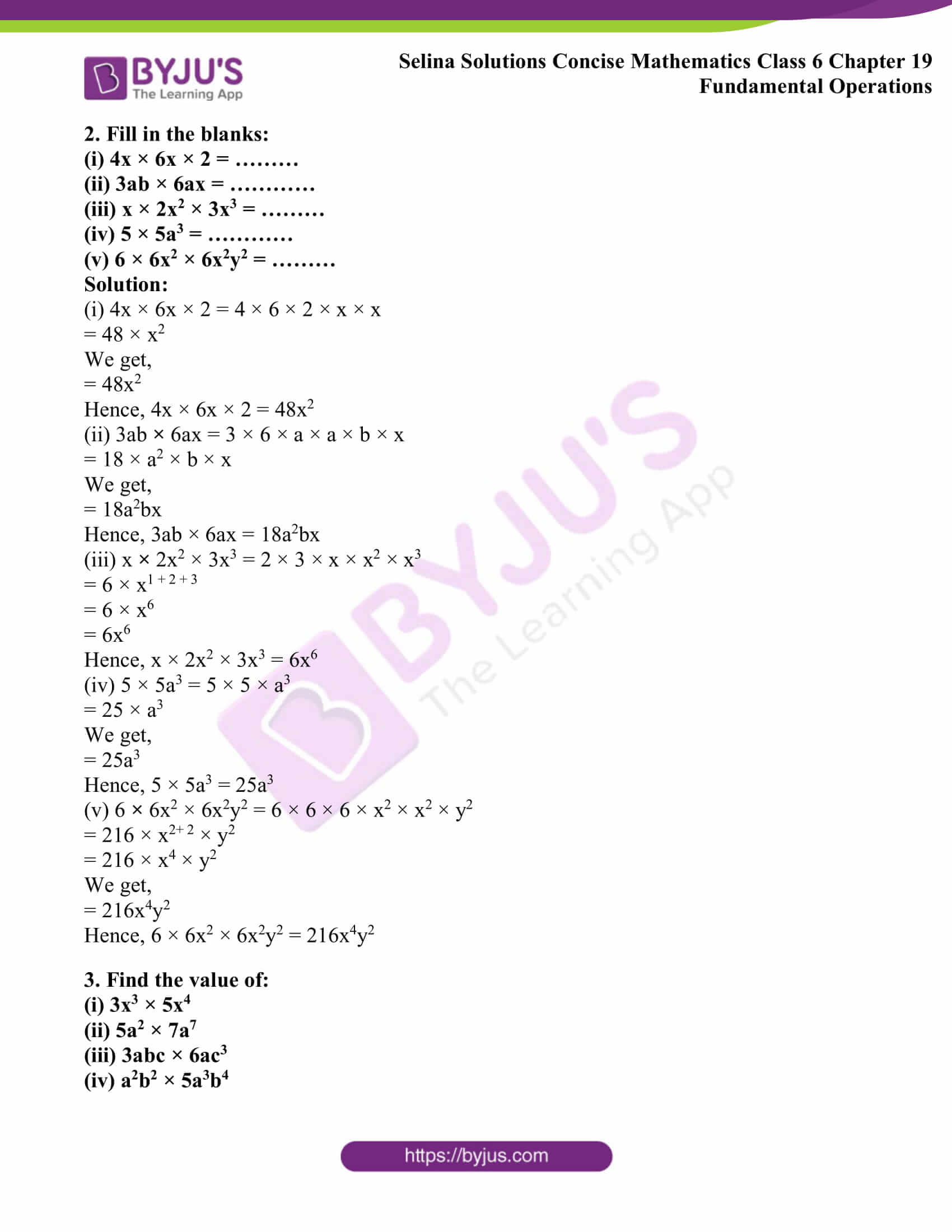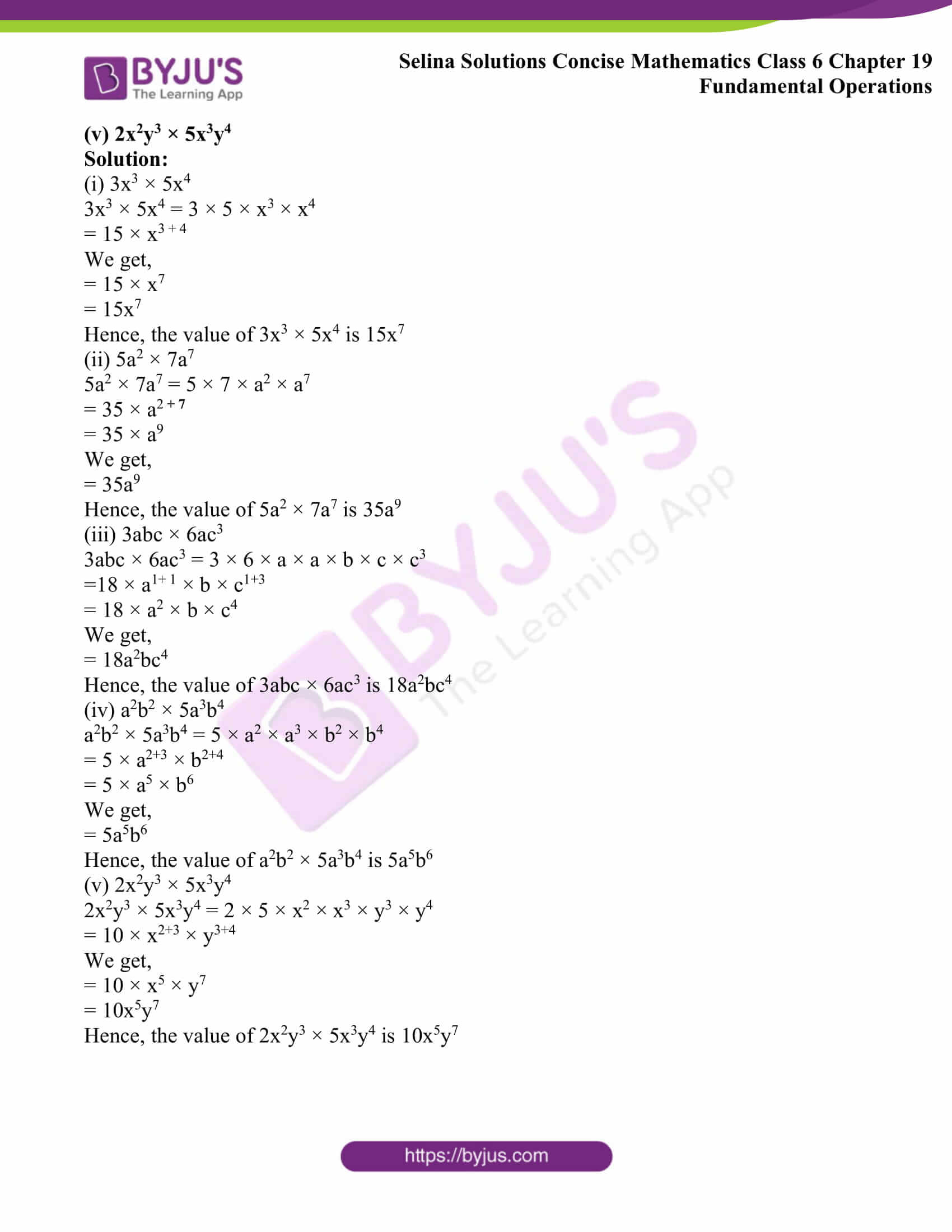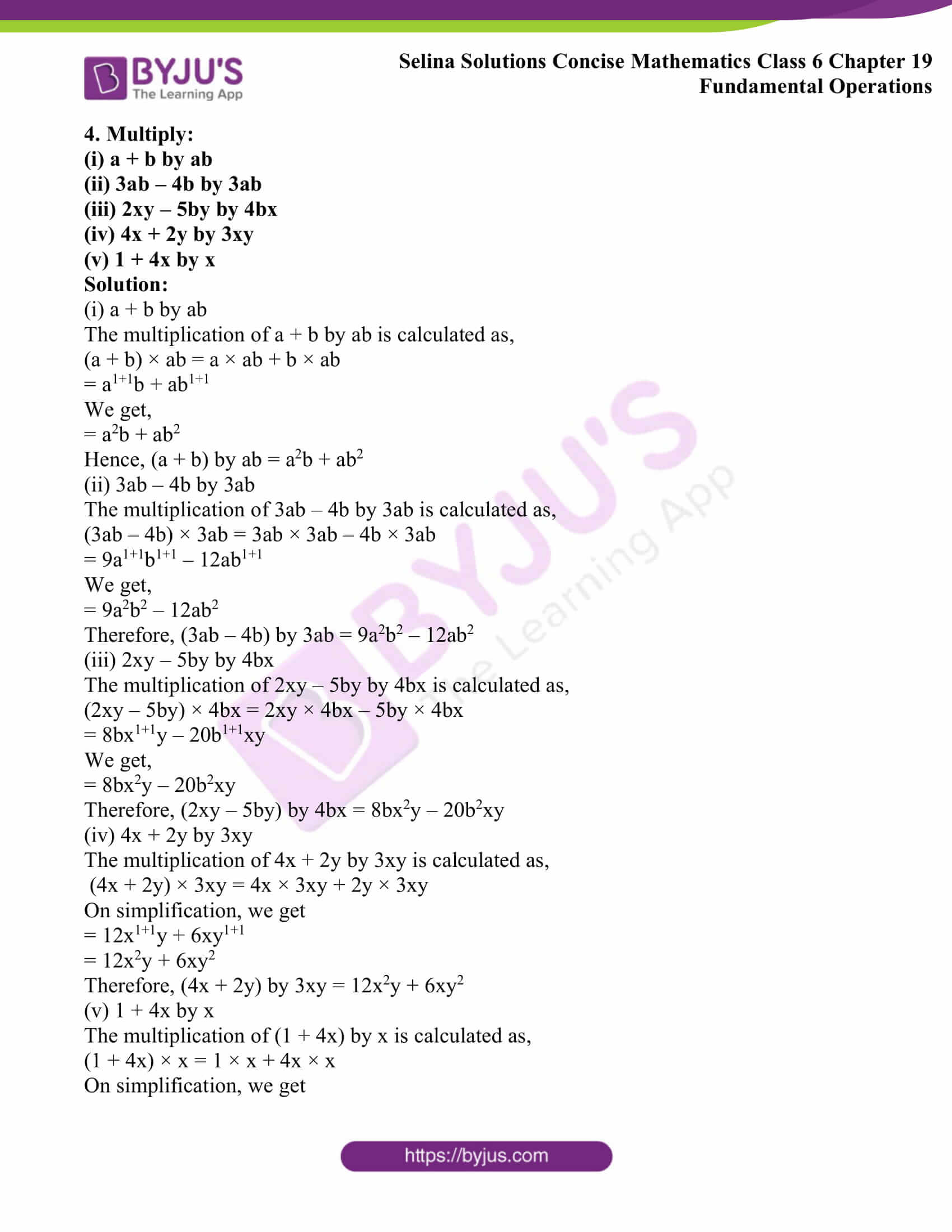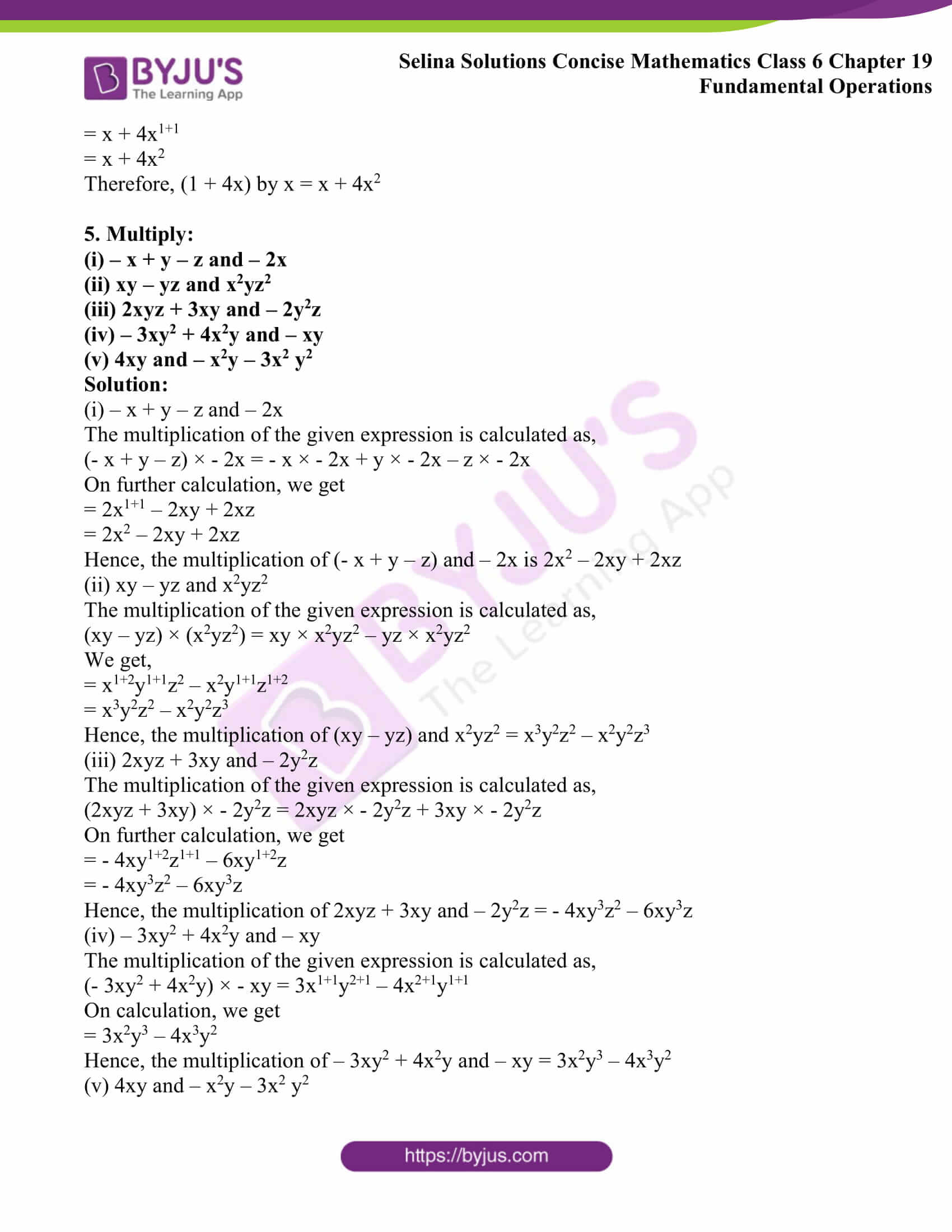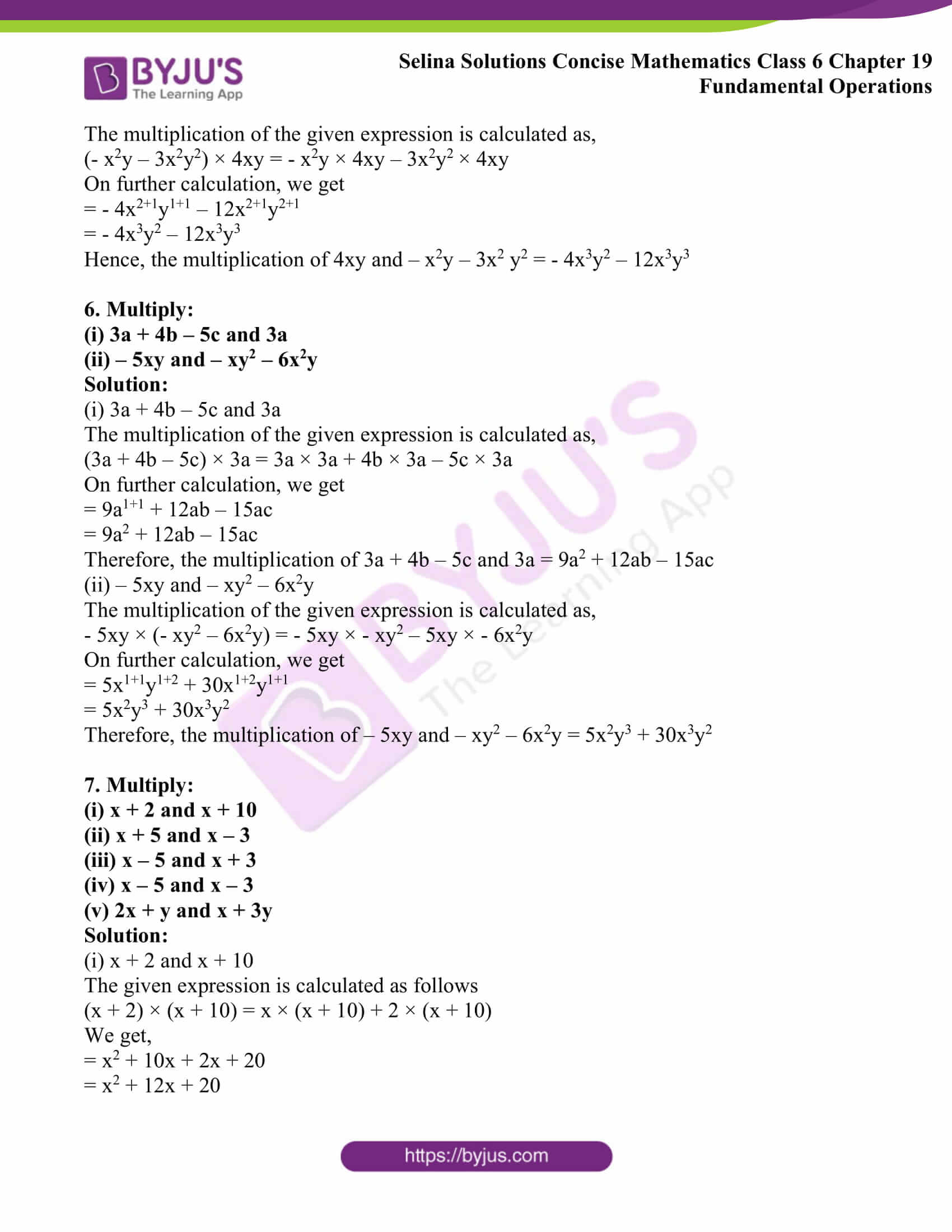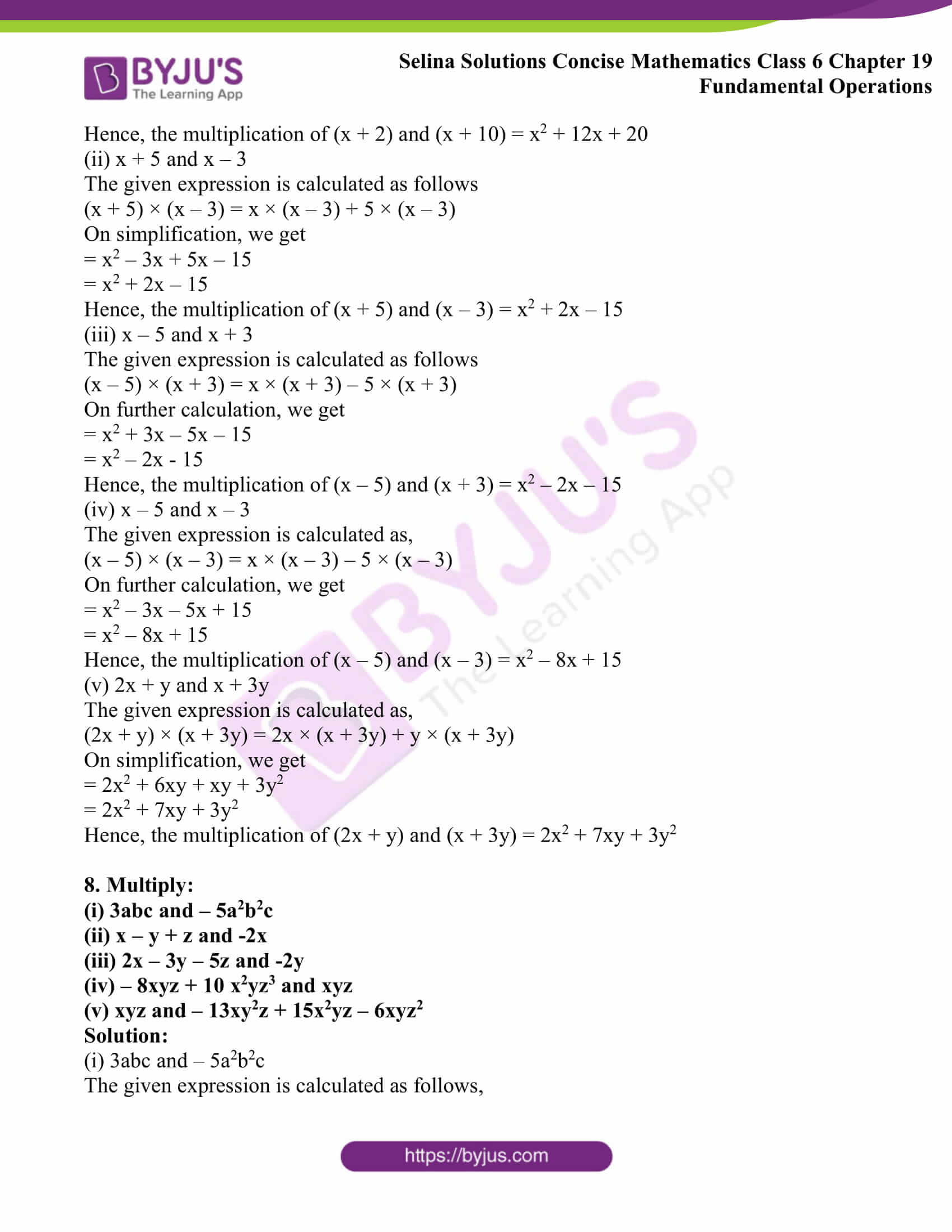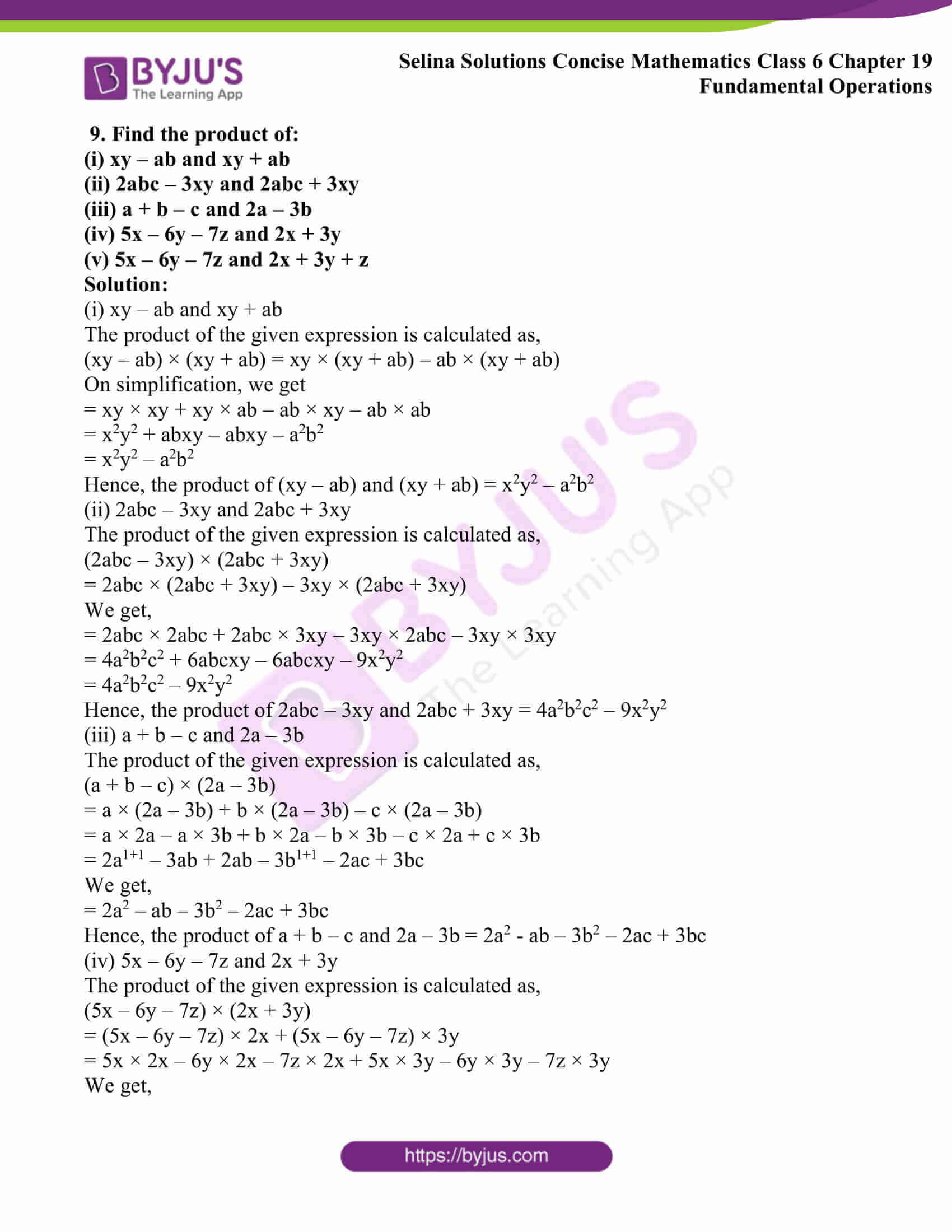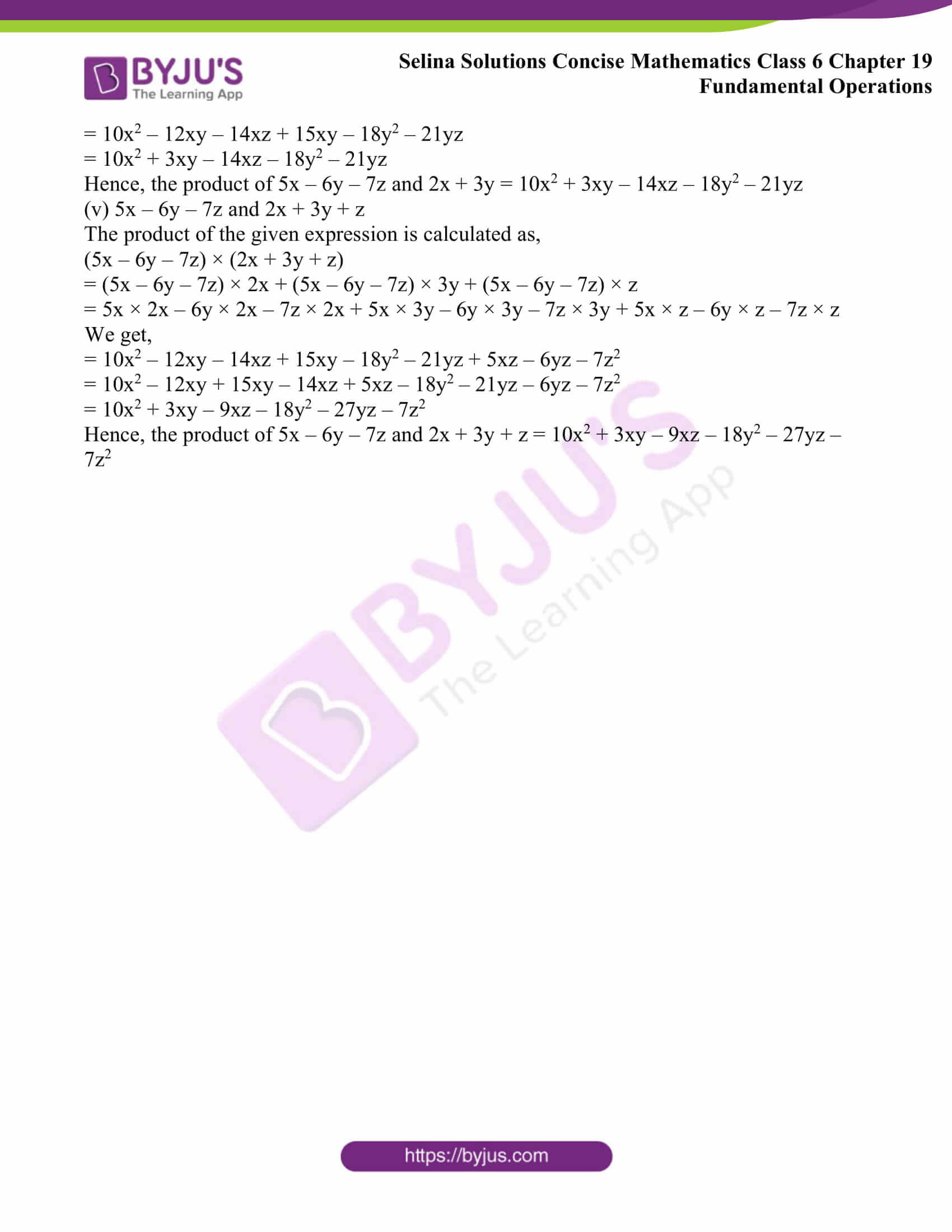### Access Selina Solutions Concise Mathematics Class 6 Chapter 19: Fundamental Operations Exercise 19(C)

Exercise 19(C)

1. Fill in the blanks:

(i) 6 × 3 = ………. and 6x × 3x = …………

(ii) 6 × 3 = ……… and 6x2 × 3x3 = …………

(iii) 5 × 4 = ………. and 5x × 4y = …………

(iv) 4 × 7 = …………. and 4ax × 7x = ……….

(v) 6 × 2 = …………. and 6xy × 2xy = ……….

Solution:

(i) 6 × 3 = 18

Hence,

6x × 3x = 6 × 3 × x × x

We get,

= 18 × x2

= 18x2

Therefore, 6 × 3 = 18 and 6x × 3x = 18x2

(ii) 6 × 3 = 18

Hence,

6x2 × 3x3 = 6 × 3 × x2 + 3

= 18 × x5

= 18x5

Therefore, 6 × 3 = 18 and 6x2 × 3x3 = 18x5

(iii) 5 × 4 = 20 and 5x × 4y = 5 × 4 × x × y

= 20xy

Therefore, 5 × 4 = 20 and 5x × 4y = 20xy

(iv) 4 × 7 = 28

Hence,

4ax × 7x = 4 × 7 × a × x × x

= 28 × a × x2

= 28ax2

Therefore, 4 × 7 = 28 and 4ax × 7x = 28ax2

(v) 6 × 2 = 12

Hence,

6xy × 2xy = 6 × 2 × x1 + 1 × y1 + 1

= 12 × x2 × y2

= 12x2y2

Therefore, 6 × 2 = 12 and 6xy × 2xy = 12x2y2

2. Fill in the blanks:

(i) 4x × 6x × 2 = ………

(ii) 3ab × 6ax = …………

(iii) x × 2x2 × 3x3 = ………

(iv) 5 × 5a3 = …………

(v) 6 × 6x2 × 6x2y2 = ………

Solution:

(i) 4x × 6x × 2 = 4 × 6 × 2 × x × x

= 48 × x2

We get,

= 48x2

Hence, 4x × 6x × 2 = 48x2

(ii) 3ab × 6ax = 3 × 6 × a × a × b × x

= 18 × a2 × b × x

We get,

= 18a2bx

Hence, 3ab × 6ax = 18a2bx

(iii) x × 2x2 × 3x3 = 2 × 3 × x × x2 × x3

= 6 × x1 + 2 + 3

= 6 × x6

= 6x6

Hence, x × 2x2 × 3x3 = 6x6

(iv) 5 × 5a3 = 5 × 5 × a3

= 25 × a3

We get,

= 25a3

Hence, 5 × 5a3 = 25a3

(v) 6 × 6x2 × 6x2y2 = 6 × 6 × 6 × x2 × x2 × y2

= 216 × x2+ 2 × y2

= 216 × x4 × y2

We get,

= 216x4y2

Hence, 6 × 6x2 × 6x2y2 = 216x4y2

3. Find the value of:

(i) 3x3 × 5x4

(ii) 5a2 × 7a7

(iii) 3abc × 6ac3

(iv) a2b2 × 5a3b4

(v) 2x2y3 × 5x3y4

Solution:

(i) 3x3 × 5x4

3x3 × 5x4 = 3 × 5 × x3 × x4

= 15 × x3 + 4

We get,

= 15 × x7

= 15x7

Hence, the value of 3x3 × 5x4 is 15x7

(ii) 5a2 × 7a7

5a2 × 7a7 = 5 × 7 × a2 × a7

= 35 × a2 + 7

= 35 × a9

We get,

= 35a9

Hence, the value of 5a2 × 7a7 is 35a9

(iii) 3abc × 6ac3

3abc × 6ac3 = 3 × 6 × a × a × b × c × c3

=18 × a1+ 1 × b × c1+3

= 18 × a2 × b × c4

We get,

= 18a2bc4

Hence, the value of 3abc × 6ac3 is 18a2bc4

(iv) a2b2 × 5a3b4

a2b2 × 5a3b4 = 5 × a2 × a3 × b2 × b4

= 5 × a2+3 × b2+4

= 5 × a5 × b6

We get,

= 5a5b6

Hence, the value of a2b2 × 5a3b4 is 5a5b6

(v) 2x2y3 × 5x3y4

2x2y3 × 5x3y4 = 2 × 5 × x2 × x3 × y3 × y4

= 10 × x2+3 × y3+4

We get,

= 10 × x5 × y7

= 10x5y7

Hence, the value of 2x2y3 × 5x3y4 is 10x5y7

4. Multiply:

(i) a + b by ab

(ii) 3ab – 4b by 3ab

(iii) 2xy – 5by by 4bx

(iv) 4x + 2y by 3xy

(v) 1 + 4x by x

Solution:

(i) a + b by ab

The multiplication of a + b by ab is calculated as,

(a + b) × ab = a × ab + b × ab

= a1+1b + ab1+1

We get,

= a2b + ab2

Hence, (a + b) by ab = a2b + ab2

(ii) 3ab – 4b by 3ab

The multiplication of 3ab – 4b by 3ab is calculated as,

(3ab – 4b) × 3ab = 3ab × 3ab – 4b × 3ab

= 9a1+1b1+1 – 12ab1+1

We get,

= 9a2b2 – 12ab2

Therefore, (3ab – 4b) by 3ab = 9a2b2 – 12ab2

(iii) 2xy – 5by by 4bx

The multiplication of 2xy – 5by by 4bx is calculated as,

(2xy – 5by) × 4bx = 2xy × 4bx – 5by × 4bx

= 8bx1+1y – 20b1+1xy

We get,

= 8bx2y – 20b2xy

Therefore, (2xy – 5by) by 4bx = 8bx2y – 20b2xy

(iv) 4x + 2y by 3xy

The multiplication of 4x + 2y by 3xy is calculated as,

(4x + 2y) × 3xy = 4x × 3xy + 2y × 3xy

On simplification, we get

= 12x1+1y + 6xy1+1

= 12x2y + 6xy2

Therefore, (4x + 2y) by 3xy = 12x2y + 6xy2

(v) 1 + 4x by x

The multiplication of (1 + 4x) by x is calculated as,

(1 + 4x) × x = 1 × x + 4x × x

On simplification, we get

= x + 4x1+1

= x + 4x2

Therefore, (1 + 4x) by x = x + 4x2

5. Multiply:

(i) – x + y – z and – 2x

(ii) xy – yz and x2yz2

(iii) 2xyz + 3xy and – 2y2z

(iv) – 3xy2 + 4x2y and – xy

(v) 4xy and – x2y – 3x2 y2

Solution:

(i) – x + y – z and – 2x

The multiplication of the given expression is calculated as,

(- x + y – z) × – 2x = – x × – 2x + y × – 2x – z × – 2x

On further calculation, we get

= 2x1+1 – 2xy + 2xz

= 2x2 – 2xy + 2xz

Hence, the multiplication of (- x + y – z) and – 2x is 2x2 – 2xy + 2xz

(ii) xy – yz and x2yz2

The multiplication of the given expression is calculated as,

(xy – yz) × (x2yz2) = xy × x2yz2 – yz × x2yz2

We get,

= x1+2y1+1z2 – x2y1+1z1+2

= x3y2z2 – x2y2z3

Hence, the multiplication of (xy – yz) and x2yz2 = x3y2z2 – x2y2z3

(iii) 2xyz + 3xy and – 2y2z

The multiplication of the given expression is calculated as,

(2xyz + 3xy) × – 2y2z = 2xyz × – 2y2z + 3xy × – 2y2z

On further calculation, we get

= – 4xy1+2z1+1 – 6xy1+2z

= – 4xy3z2 – 6xy3z

Hence, the multiplication of 2xyz + 3xy and – 2y2z = – 4xy3z2 – 6xy3z

(iv) – 3xy2 + 4x2y and – xy

The multiplication of the given expression is calculated as,

(- 3xy2 + 4x2y) × – xy = 3x1+1y2+1 – 4x2+1y1+1

On calculation, we get

= 3x2y3 – 4x3y2

Hence, the multiplication of – 3xy2 + 4x2y and – xy = 3x2y3 – 4x3y2

(v) 4xy and – x2y – 3x2 y2

The multiplication of the given expression is calculated as,

(- x2y – 3x2y2) × 4xy = – x2y × 4xy – 3x2y2 × 4xy

On further calculation, we get

= – 4x2+1y1+1 – 12x2+1y2+1

= – 4x3y2 – 12x3y3

Hence, the multiplication of 4xy and – x2y – 3x2 y2 = – 4x3y2 – 12x3y3

6. Multiply:

(i) 3a + 4b – 5c and 3a

(ii) – 5xy and – xy2 – 6x2y

Solution:

(i) 3a + 4b – 5c and 3a

The multiplication of the given expression is calculated as,

(3a + 4b – 5c) × 3a = 3a × 3a + 4b × 3a – 5c × 3a

On further calculation, we get

= 9a1+1 + 12ab – 15ac

= 9a2 + 12ab – 15ac

Therefore, the multiplication of 3a + 4b – 5c and 3a = 9a2 + 12ab – 15ac

(ii) – 5xy and – xy2 – 6x2y

The multiplication of the given expression is calculated as,

– 5xy × (- xy2 – 6x2y) = – 5xy × – xy2 – 5xy × – 6x2y

On further calculation, we get

= 5x1+1y1+2 + 30x1+2y1+1

= 5x2y3 + 30x3y2

Therefore, the multiplication of – 5xy and – xy2 – 6x2y = 5x2y3 + 30x3y2

7. Multiply:

(i) x + 2 and x + 10

(ii) x + 5 and x – 3

(iii) x – 5 and x + 3

(iv) x – 5 and x – 3

(v) 2x + y and x + 3y

Solution:

(i) x + 2 and x + 10

The given expression is calculated as follows

(x + 2) × (x + 10) = x × (x + 10) + 2 × (x + 10)

We get,

= x2 + 10x + 2x + 20

= x2 + 12x + 20

Hence, the multiplication of (x + 2) and (x + 10) = x2 + 12x + 20

(ii) x + 5 and x – 3

The given expression is calculated as follows

(x + 5) × (x – 3) = x × (x – 3) + 5 × (x – 3)

On simplification, we get

= x2 – 3x + 5x – 15

= x2 + 2x – 15

Hence, the multiplication of (x + 5) and (x – 3) = x2 + 2x – 15

(iii) x – 5 and x + 3

The given expression is calculated as follows

(x – 5) × (x + 3) = x × (x + 3) – 5 × (x + 3)

On further calculation, we get

= x2 + 3x – 5x – 15

= x2 – 2x – 15

Hence, the multiplication of (x – 5) and (x + 3) = x2 – 2x – 15

(iv) x – 5 and x – 3

The given expression is calculated as,

(x – 5) × (x – 3) = x × (x – 3) – 5 × (x – 3)

On further calculation, we get

= x2 – 3x – 5x + 15

= x2 – 8x + 15

Hence, the multiplication of (x – 5) and (x – 3) = x2 – 8x + 15

(v) 2x + y and x + 3y

The given expression is calculated as,

(2x + y) × (x + 3y) = 2x × (x + 3y) + y × (x + 3y)

On simplification, we get

= 2x2 + 6xy + xy + 3y2

= 2x2 + 7xy + 3y2

Hence, the multiplication of (2x + y) and (x + 3y) = 2x2 + 7xy + 3y2

8. Multiply:

(i) 3abc and – 5a2b2c

(ii) x – y + z and -2x

(iii) 2x – 3y – 5z and -2y

(iv) – 8xyz + 10 x2yz3 and xyz

(v) xyz and – 13xy2z + 15x2yz – 6xyz2

Solution:

(i) 3abc and – 5a2b2c

The given expression is calculated as follows,

3abc × – 5a2b2c = 3 × – 5 × a × a2 × b × b2 × c × c

On further calculation, we get

= – 15 × a1+2 × b1+2 × c1+1

= – 15 × a3 × b3 × c2

= – 15a3b3c2

Therefore, the multiplication of 3abc and – 5a2b2c = – 15a3b3c2

(ii) x – y + z and -2x

The given expression is calculated as follows,

(x – y + z) × – 2x = x × – 2x – y × – 2x + z × – 2x

On simplification, we get

= – 2x1+1 + 2xy – 2xz

= – 2x2 + 2xy – 2xz

Therefore, the multiplication of x – y + z and -2x = – 2x2 + 2xy – 2xz

(iii) 2x – 3y – 5z and -2y

The given expression is calculated as follows,

(2x – 3y – 5z) × – 2y = 2x × – 2y – 3y × – 2y – 5z × – 2y

On further calculation, we get

= – 4xy + 6y1+1 + 10yz

= – 4xy + 6y2 + 10yz

Therefore, the multiplication of 2x – 3y – 5z and -2y = – 4xy + 6y2 + 10yz

(iv) – 8xyz + 10 x2yz3 and xyz

The given expression is calculated as follows,

(- 8xyz + 10x2yz3) × xyz = – 8xyz × xyz + 10x2yz3 × xyz

On further calculation, we get

= – 8x1+1y1+1z1+1 + 10x2+1y1+1z3+1

= – 8x2y2z2 + 10x3y2z4

Therefore, the multiplication of – 8xyz + 10 x2yz3 and xyz = – 8x2y2z2 + 10x3y2z4

(v) xyz and – 13xy2z + 15x2yz – 6xyz2

The given expression is calculated as follows,

xyz × (- 13xy2z + 15x2yz – 6xyz2) = xyz × – 13xy2z + xyz ×15x2yz – xyz × 6xyz2

On simplification, we get

= – 13x1+1y1+2z1+1 + 15x1+2y1+1z1+1 – 6x1+1y1+1z1+2

We get,

= – 13x2y3z2 + 15x3y2z2 – 6x2y2z3

Therefore, the multiplication of xyz and – 13xy2z + 15x2yz – 6xyz2 = – 13x2y3z2 + 15x3y2z2 – 6x2y2z3

9. Find the product of:

(i) xy – ab and xy + ab

(ii) 2abc – 3xy and 2abc + 3xy

(iii) a + b – c and 2a – 3b

(iv) 5x – 6y – 7z and 2x + 3y

(v) 5x – 6y – 7z and 2x + 3y + z

Solution:

(i) xy – ab and xy + ab

The product of the given expression is calculated as,

(xy – ab) × (xy + ab) = xy × (xy + ab) – ab × (xy + ab)

On simplification, we get

= xy × xy + xy × ab – ab × xy – ab × ab

= x2y2 + abxy – abxy – a2b2

= x2y2 – a2b2

Hence, the product of (xy – ab) and (xy + ab) = x2y2 – a2b2

(ii) 2abc – 3xy and 2abc + 3xy

The product of the given expression is calculated as,

(2abc – 3xy) × (2abc + 3xy)

= 2abc × (2abc + 3xy) – 3xy × (2abc + 3xy)

We get,

= 2abc × 2abc + 2abc × 3xy – 3xy × 2abc – 3xy × 3xy

= 4a2b2c2 + 6abcxy – 6abcxy – 9x2y2

= 4a2b2c2 – 9x2y2

Hence, the product of 2abc – 3xy and 2abc + 3xy = 4a2b2c2 – 9x2y2

(iii) a + b – c and 2a – 3b

The product of the given expression is calculated as,

(a + b – c) × (2a – 3b)

= a × (2a – 3b) + b × (2a – 3b) – c × (2a – 3b)

= a × 2a – a × 3b + b × 2a – b × 3b – c × 2a + c × 3b

= 2a1+1 – 3ab + 2ab – 3b1+1 – 2ac + 3bc

We get,

= 2a2 – ab – 3b2 – 2ac + 3bc

Hence, the product of a + b – c and 2a – 3b = 2a2 – ab – 3b2 – 2ac + 3bc

(iv) 5x – 6y – 7z and 2x + 3y

The product of the given expression is calculated as,

(5x – 6y – 7z) × (2x + 3y)

= (5x – 6y – 7z) × 2x + (5x – 6y – 7z) × 3y

= 5x × 2x – 6y × 2x – 7z × 2x + 5x × 3y – 6y × 3y – 7z × 3y

We get,

= 10x2 – 12xy – 14xz + 15xy – 18y2 – 21yz

= 10x2 + 3xy – 14xz – 18y2 – 21yz

Hence, the product of 5x – 6y – 7z and 2x + 3y = 10x2 + 3xy – 14xz – 18y2 – 21yz

(v) 5x – 6y – 7z and 2x + 3y + z

The product of the given expression is calculated as,

(5x – 6y – 7z) × (2x + 3y + z)

= (5x – 6y – 7z) × 2x + (5x – 6y – 7z) × 3y + (5x – 6y – 7z) × z

= 5x × 2x – 6y × 2x – 7z × 2x + 5x × 3y – 6y × 3y – 7z × 3y + 5x × z – 6y × z – 7z × z

We get,

= 10x2 – 12xy – 14xz + 15xy – 18y2 – 21yz + 5xz – 6yz – 7z2

= 10x2 – 12xy + 15xy – 14xz + 5xz – 18y2 – 21yz – 6yz – 7z2

= 10x2 + 3xy – 9xz – 18y2 – 27yz – 7z2

Hence, the product of 5x – 6y – 7z and 2x + 3y + z = 10x2 + 3xy – 9xz – 18y2 – 27yz – 7z2